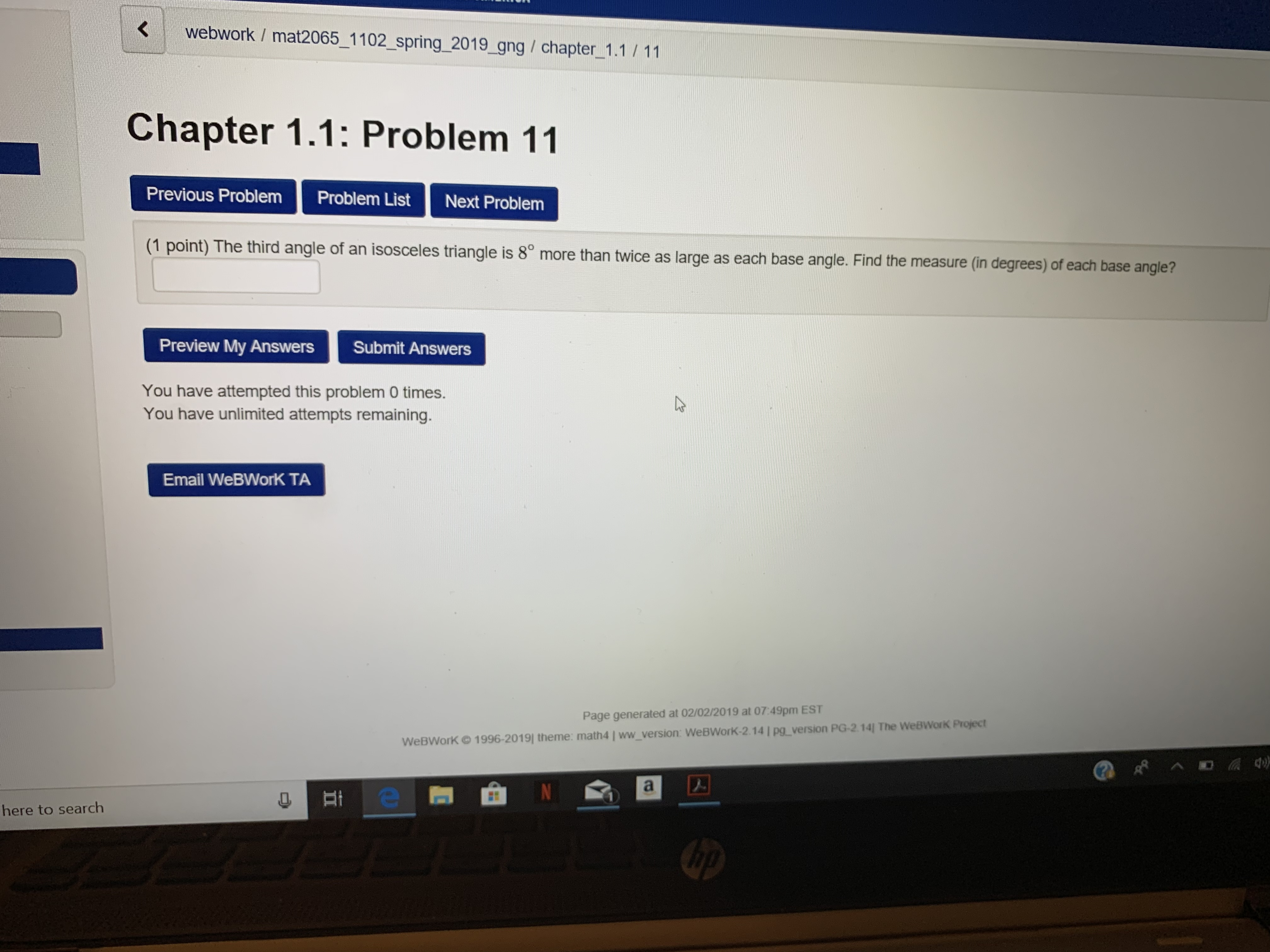Kwebwork / mat2065_1102_spring_2019 gng / chapter 1.1 11Chapter 1.1: Problem 11Previous ProblemProblem ListNext Problem(1 point) The third angle of an isosceles triangle is 8° more than twice as large as each base angle. Fles triangle is 8° more than twice as large as each base angle. Find the measure (in degrees) of each base angle?Preview My AnswersSubmit AnswersYou have attempted this problem 0 times.You have unlimited attempts remainingEmail WeBWorK TAPage generated at 02/02/2019 at 07:49pm ESTWeBWorK © 1996-2019( theme math41 ww version WeBWorK-2 141 pa-version PG-2 14] The WeBworK Proyecthere to search

Questionhelp_outlineImage TranscriptioncloseKwebwork / mat2065_1102_spring_2019 gng / chapter 1.1 11 Chapter 1.1: Problem 11 Previous Problem Problem List Next Problem (1 point) The third angle of an isosceles triangle is 8° more than twice as large as each base angle. F les triangle is 8° more than twice as large as each base angle. Find the measure (in degrees) of each base angle? Preview My Answers Submit Answers You have attempted this problem 0 times. You have unlimited attempts remaining Email WeBWorK TA Page generated at 02/02/2019 at 07:49pm EST WeBWorK © 1996-2019( theme math41 ww version WeBWorK-2 141 pa-version PG-2 14] The WeBworK Proyect here to search fullscreen
Step 1

Since base angle of isosceles triangle is equal so let each base angle = x

So twice of the base angle = 2x

And third angle = 2x + 8

Step 2

Now three angles of given isosceles triangle are x, x, 2x+8

Step 3

Using angle sum property of triangle

x + x + 2x + 8 = 180

4x + 8 ...

Want to see the full answer?

See Solution

Want to see this answer and more?

Our solutions are written by experts, many with advanced degrees, and available 24/7

See Solution
Tagged in

Equations and In-equations